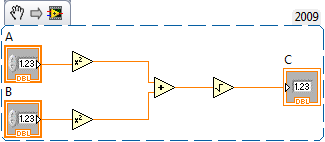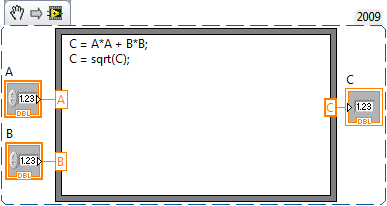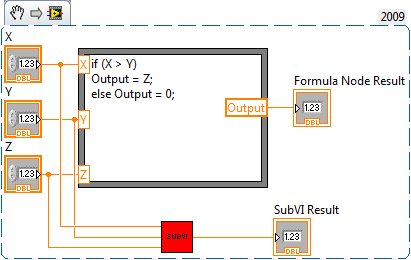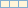# Curriculum and Labs for Engineering Education

cancel
Showing results for
Did you mean:

## Unit 2 - Fundamentals: Lesson 1

Introduction

In this lesson we are going to learn about SubVIs and forumla nodes.

SubVIs are similar to subroutines from other programming langauges, in that they let us encapsulate code. SubVIs are similar to other VIs that we have created. But, SubVIs are executed from within another VI. Formula nodes on the other hand allow users to implement equations using text based instructions.

Lesson Video 1

Example SnippetLesson Video 2

Example SnippetPractice Problem

Procedure: Create the required VIs to achieve the follwoing tasks.

Requirements:

1. Create a formula node which has 3 inputs(X, Y, Z) and one output. If X>Y output Z, otherwise output 0.
2. Create a SubVI which performs the same task as the formula node. (Hint: Use the selection function found in the comparison palette)

Practice SolutionNext Step

Unit 2 - Fundamentals: Lesson 2